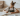# Mastering the Area of Triangles, Its Formula, and Applications

POSTED ON MARCH 23, 2023It’s time to know the different ways to calculate the triangle’s area. From using its base and height to the triangle’s three sides, you’ll find the answer you’re looking for in this article!

## What Is the Formula for the Area of Triangles?

The simplest formula for the triangle’s area is 1/2 x b x h, where b and h is the triangle’s base and height, respectively. If you’ve watched the video, you’ll see altitude. Don’t worry, altitude and height both mean the same! This means that given the triangle’s base and height, all you have to do is multiply both and then divide the resulting value by 2.When given the triangle’s base and height, its area is just equal to half the product of the base and its height. Take a look at the triangle with a base of 12 inches and a height of 10 inches as shown below.The area of the triangle is simply equal to half the product of 12 inches and 10 inches. After watching the video, you’ll know that the triangle’s area measures the amount of space within the triangle. Once you have the triangle’s area, ensure to finalize your answer in squared units or in this case, in squared inches. Hence, the triangle’s area is equal to 1/2 x 12 x 10 = 60 in².

#### Example 1

The best way to master this formula is by working on different examples yourself. When you’re ready, calculate the areas of the figures shown below.A. Focus on the triangular region of the holiday hat: it has a height of 9 inches and a base of 6 inches. To calculate its area, take half the product of the base and height.Hence, the area of the red triangular section is equal to 1/2 x 6 x 9 = 27 in².

B. For the second figure, consider the external height formed by the vertex of the triangle with the base. As long as the two lines form a right angle, consider the external base as the triangle’s base.This means that the area of the triangular signage is equal to 1/2 x 8 x 12 = 48 cm². Now that you’re all warmed up and ready to learn more about triangles and their areas, it’s time to focus on finding the area of a right triangle.

Boost math confidence to the next level
Juni’s vetted instructors study at top US Universities and provide our students with the support and mentorship to grow their math skills.

## How to Calculate the Area of a Right Triangle?

To calculate the area of a right triangle, apply the same process: take half of the product between the right triangle’s height and base. If the height is not given, use the Pythagorean theorem, c² = a² + b² , where c represents the hypotenuse, a represents the base, and b represents the right triangle’s height.

Juni Learning has excellent resources for exploring the new landscape of math learning and comprehension. If you’d like to encourage more interaction with lessons in Common Core’s mathematical standards, visit Juni Learning to explore their options. Just like those math standards, Juni Learning’s catalog can encourage exploration in other subjects, too!#### Example 2

Take a look at the right triangle shown below. Use what you’ve just learned to find its area.The right triangle’s hypotenuse and base are given. However, its height’s measure is missing. Use the Pythagorean theorem to calculate the right triangle’s height. Replace the hypotenuse and base’ measures into the formula to solve for the height, h.Once you have both the base and height of the right triangle, apply the formula for the triangle’s area. Hence, the right triangle’s area is equal to 1/2 x 12 x 16 = 96 cm².

We’ve covered all the fundamental bases, so it’s time to ask even more challenging questions.

Our advisors can answer any questions you have about our curriculum or subscriptions. They can even provide a course recommendation.

## How to Calculate the Triangle’s Area Given All Three Sides?

Use Heron’s formula to find the triangle’s area given the lengths of all three sides. Why can’t we just use the area’s basic formula? That’s because the height is not given and there’s no guarantee that we can easily find the triangle’s height. This is where Heron’s formula comes in handy!Heron’s formula highlights the fact that it’s possible to find the triangle’s area given its three sides. Suppose that a, b, and c are the three sides of the triangle and s represents half the triangle’s perimeter, its area is equal to the equation pictured above.

STEP 1: Find the value of s by taking half of the triangle’s perimeter (watch this video for a refresher).STEP 2: Calculate the area by applying Heron’s formula.#### Example 3

Test your understanding by calculating the triangle’s area shown below.First, calculate the perimeter of the triangle then take half of it to find the value of s.

s = (12+12 +10)/2 = 34 m

Apply Heron’s formula to calculate the triangle’s area as shown below.Hence, the triangle’s area is approximately equal to 54.54 m².